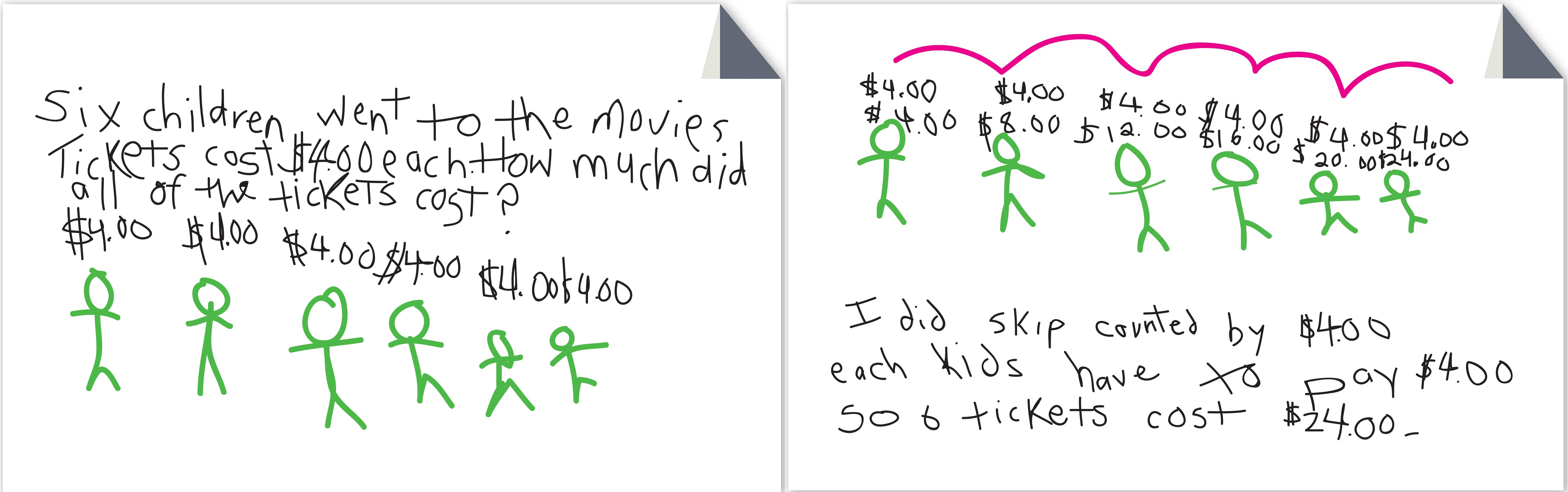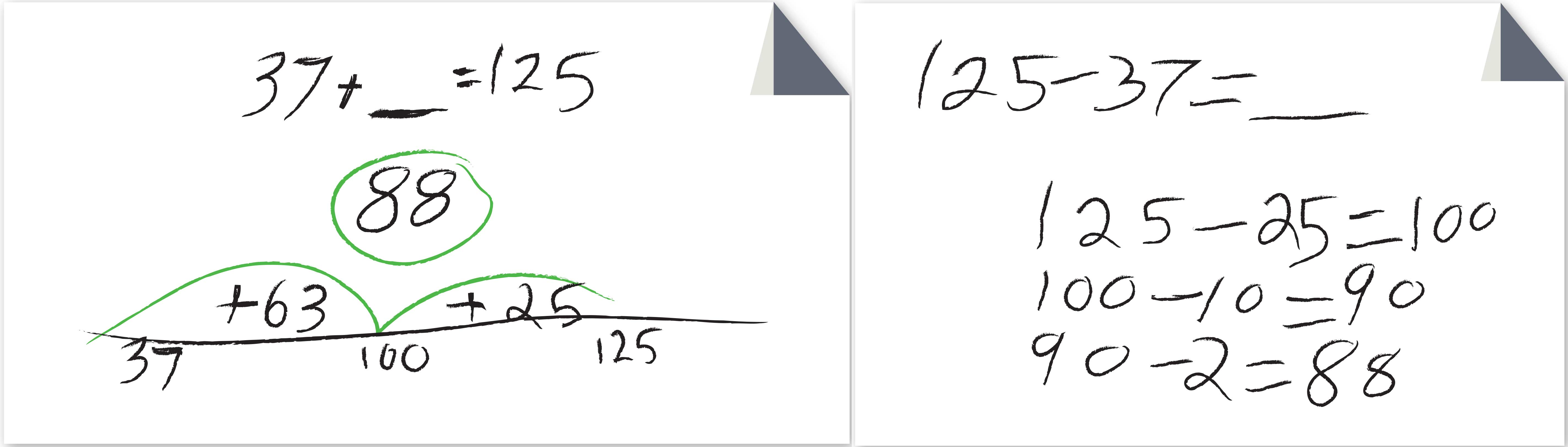Select Page

Answer: We often hear from people who wonder why Grade 3 starts with a multiplication and division unit—just like it did in the 1st edition!—rather than an addition and subtraction unit. As we decided on the sequence of the units in any grade, we considered many different things. The most important was the development of mathematical content within and across grades.

In Investigations 3, multiplication and division is a major focus in Grade 3. We wanted 3rd graders to start the year with the important work of the grade and to begin developing a conceptual understanding of multiplication and division. Also, there are three units focused on multiplication and division; putting one first allowed us to spread them sensibly across the year. (The other units are Units 5 and 8.)Understanding Equal Groups is a great way to start the year as it provides a level playing field. Ideas about multiplication and division are, for the most part, new for all students. The ideas are accessible to all, and most students get excited about starting the year digging into new mathematical ideas. In contrast, if the year starts with addition and subtraction, most students already have a history with these operations, and for some it has not been positive. Others may understand the concepts of addition and subtraction, but begin to struggle when they are asked to add and subtract larger numbers.

In fact, putting the 1st addition and subtraction unit 3rd in the sequence allowed us to include review of addition, subtraction, and place value ideas across Units 1 and 2, in the Ten-Minute Math Activity Practicing Place Value and through the Daily Practice Pages. Our intent was to provide teachers the opportunity to observe students doing this work, and enable them to identify those who might need additional support to be ready for Unit 3, which assumes students can fluently add and subtract within 100 and use representations to add and subtract within 1,000.

The question about why start the year with multiplication and division seems to suggest that third graders should work on addition and subtraction before multiplication and division. While 8- and 9-year olds are likely more familiar with addition and subtraction, there’s no reason it needs to come first.

Understanding Equal Groups focuses on the meaning of multiplication and division. The numbers are small, generally less than 100. Students use contexts and representations as they begin to develop strategies for solving multiplication and division problems. The focus is on coming to understand and visualize these operations through constructing equal groups or arrays. Later, students will build on this foundation as they develop efficient strategies. While Travel Stories and Collections is about understanding the operations of addition and subtraction, it’s much more about extending students’ understanding of those operations, especially subtraction, and beginning to refine addition and subtraction strategies. It’s a big difference.By now, 3rd grade teachers have finished Unit 1. We’d be interested in hearing how it went!

We are excited to have a space that offers us the opportunity to answer common questions from the field. Have questions you’d like to see answered? Email us.

Tag(s): addition/subtraction | grade 3 | multiplication/division | Q&A | Unit Sequence |